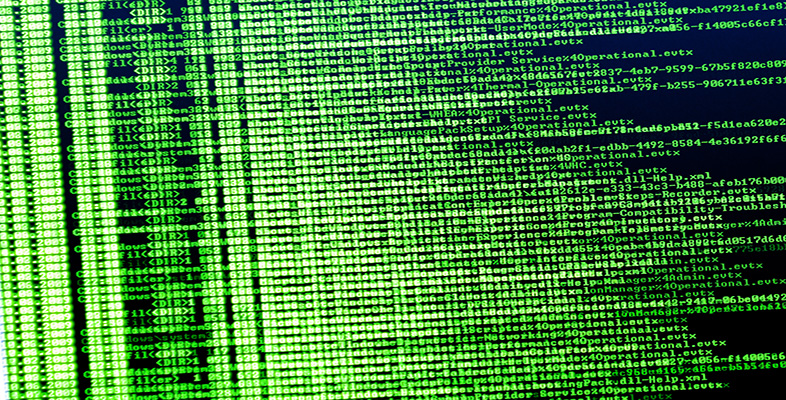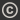Science, Maths & Technology
Free course

# Exploring data: Graphs and numerical summaries

Free statement of participation on completion## Course reviews

This free course, Exploring data: graphs and numerical summaries, will introduce you to a number of ways of representing data graphically and of summarising data numerically. You will learn the uses for pie charts, bar charts, histograms and scatterplots. You will also be introduced to various ways of summarising data and methods for assessing location and dispersion.

## Course learning outcomes

After studying this course, you should be able to:

• understand and use standard symbols and notation: for the pth value in a data set when the values are written in order, the sample lower and upper quartiles and the sample median, the sample mean and the standard deviation
• understand that data can have a pattern which may be represented graphically
• understand that the standard deviation and the interquartile range are measures of the dispersion in a data set
• understand that the median and the interquartile range are more resistant measures than are the mean and the standard deviation
• identify an overall 'feel' for data and the way it is distributed by constructing appropriate graphical displays.

First Published: 10/08/2012

Updated: 30/11/2018

Skip Rate and Review